# SAT II Math I : Distance Formula

## Example Questions

### Example Question #1 : Distance Formula

Find the distance between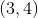and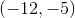.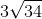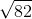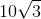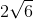Explanation:

For this problem we will need to use the distance formula: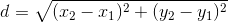In our case,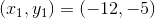and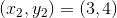.

Plugging these values into the formula we are able to find the distance.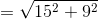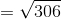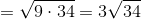### Example Question #1 : Distance Formula

What is the distance between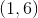and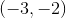?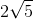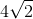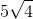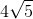Explanation:

Write the distance formula.Substitute the points: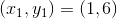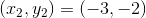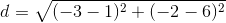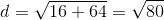The radical can be broken down into factors of perfect squares.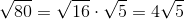The answer is: